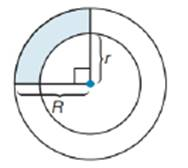Chapter 8.5, Problem 34EElementary Geometry For College St...

7th Edition
Alexander + 2 others
ISBN: 9781337614085

Solutions

Chapter
SectionElementary Geometry For College St...

7th Edition
Alexander + 2 others
ISBN: 9781337614085
Textbook Problem

Find a formula for the area of the shaded region, which represents one-fourth of an annulus (ring).To determine

To find:

A formula for area of shaded region.

Explanation

Formula:

Area of circle:

If r is the radius of circle, then area of circle is given by the formula:

Acircle=πr2.

Calculation:

To find the area of shaded region, let’s find the area of annulus (ring) between the two circles.

The area of ring between the two circles can be determined by subtracting the area of inner circle from the area of outer circle.

Aring=Aouter circle-Ainner circle

The radius of outer circle is R.

So, the area of outer circle = πR2

The radius of inner circle is r.

So, the area of inner circle = πr2

Aring=Aouter circle-Ainner ci

Still sussing out bartleby?

Check out a sample textbook solution.

See a sample solution

The Solution to Your Study Problems

Bartleby provides explanations to thousands of textbook problems written by our experts, many with advanced degrees!

Get Started

Evaluate the expression sin Exercises 116. 23

Finite Mathematics and Applied Calculus (MindTap Course List)

Polynomial Inequalities Solve the inequality. 95. x4 7x2 18 0

Precalculus: Mathematics for Calculus (Standalone Book)

Write the number in the form a + bi. 42. e2i

Single Variable Calculus: Early Transcendentals, Volume I

In Problems 11-20, find the indicated derivatives and simplify. 13.

Mathematical Applications for the Management, Life, and Social Sciences

Multiply the following numbers. 22.

Contemporary Mathematics for Business & Consumers